﻿ 基于数值仿真与经验公式的船舶破冰阻力预报
 舰船科学技术2019, Vol. 41Issue (8): 1-6PDF

1. 江苏科技大学 船舶与海洋工程学院，江苏 镇江 212003;
2. 江苏新扬子造船有限公司，江苏 泰州 214500

Prediction of ice breaking resistance of ship based on numerical simulation and empirical formulation
ZHANG Jian1, WANG Kai-min1, XU Jun-hua1, DU Cheng-zhong2
1. School of Navy Architecture and Ocean Engineering, Jiangsu University of Science and Technology, Zhenjiang 212003, China;
2. Jiangsu New Yangzi Shipbuilding Co., Ltd., Taizhou 214500, China
Abstract: Firstly, the appropriate ice material model was selected and verified. By establishing the relevant finite element model of level ice and icebreaker, the continuous icebreaking process of icebreaker under different ice thickness and different ship speeds was implemented by using nonlinear explicit power software Ls-dyna. The resistance of ice calculated from numerical simulation results and empirical formulas which were compared under different ice conditions. Finally, the sensitivity of the main parameters in each empirical formulation was analyzed, and the degree of difference in the impact of each parameter on the ice resistance calculation was obtained, which is of important reference value for ice resistance estimation and related hull structural design.
Key words: icebreaker     ice resistance     numerical simulation     empirical formulation
0 引　言

1 冰材料及模型验证表 1 冰材料参数 Tab.1 The material parameters of ice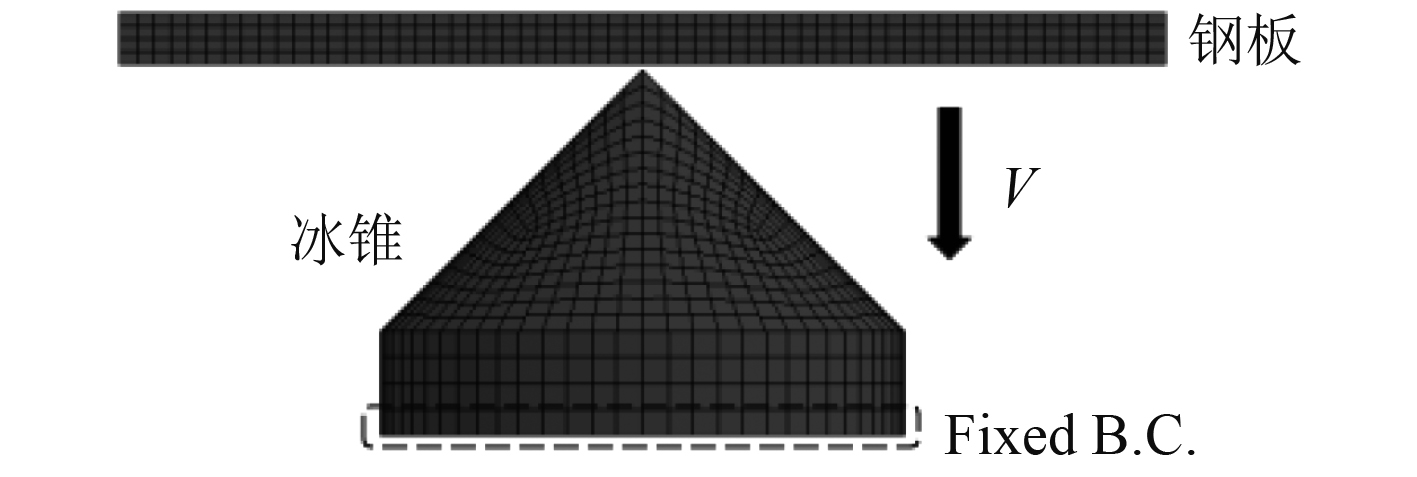图 1 钢板与冰锥模型 Fig. 1 FE model of ice cone and steel plate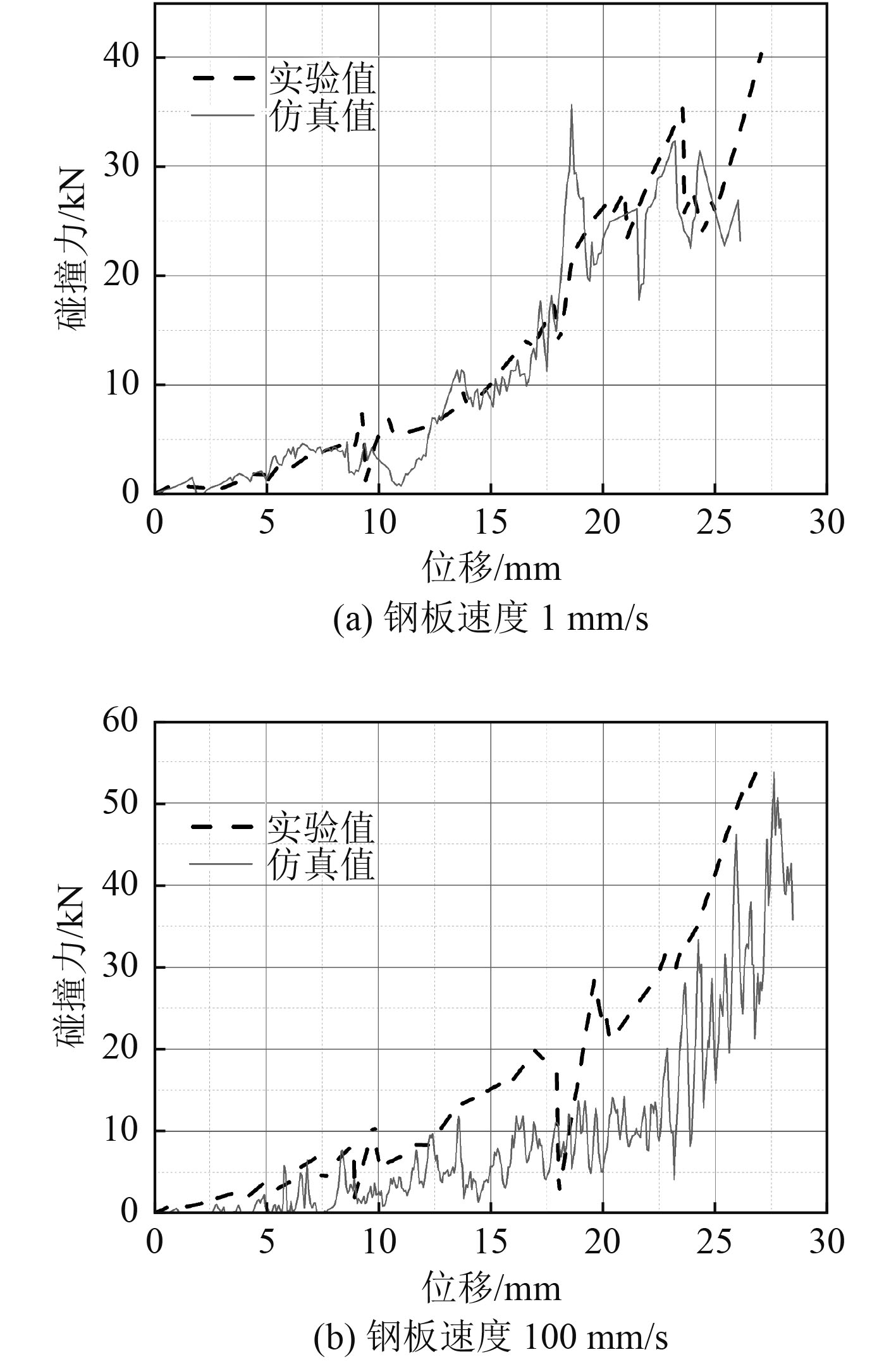图 2 钢板不同运动速度下的冰力-位移曲线 Fig. 2 Curve of ice force-displacement at different steel plate speeds

2 破冰船连续式破冰数值模拟 2.1 破冰船与冰排模型表 2 极地破冰船材料参数 Tab.2 Polar icebreaker material parameters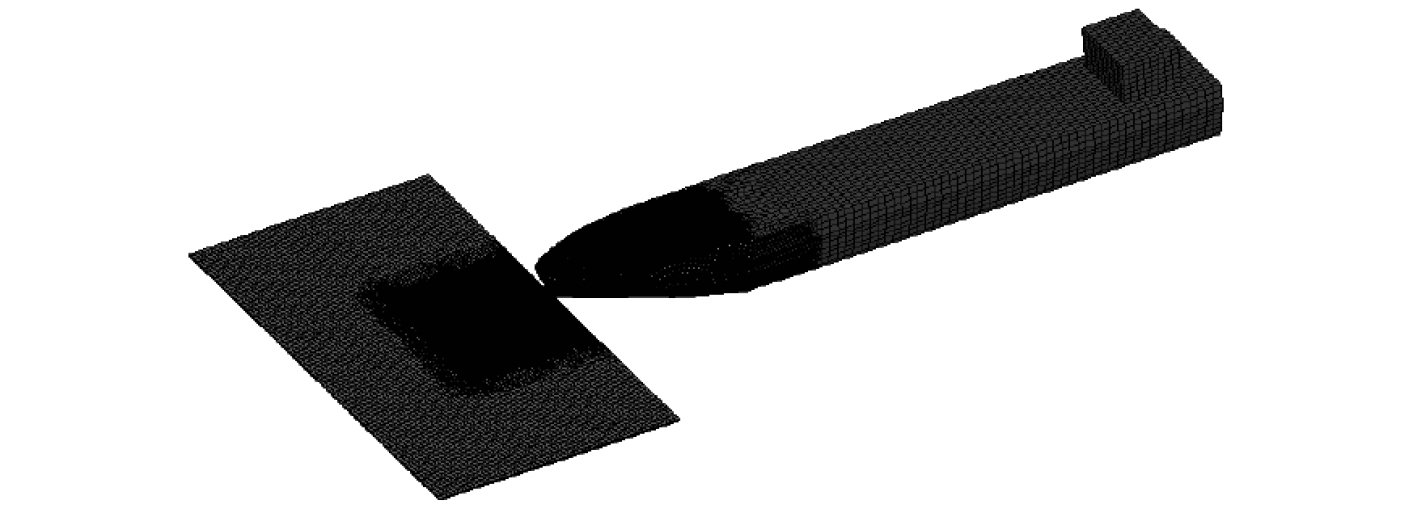图 3 破冰船和冰排模型 Fig. 3 The model of icebreaker and ice row
2.2 船速和冰厚对冰阻力影响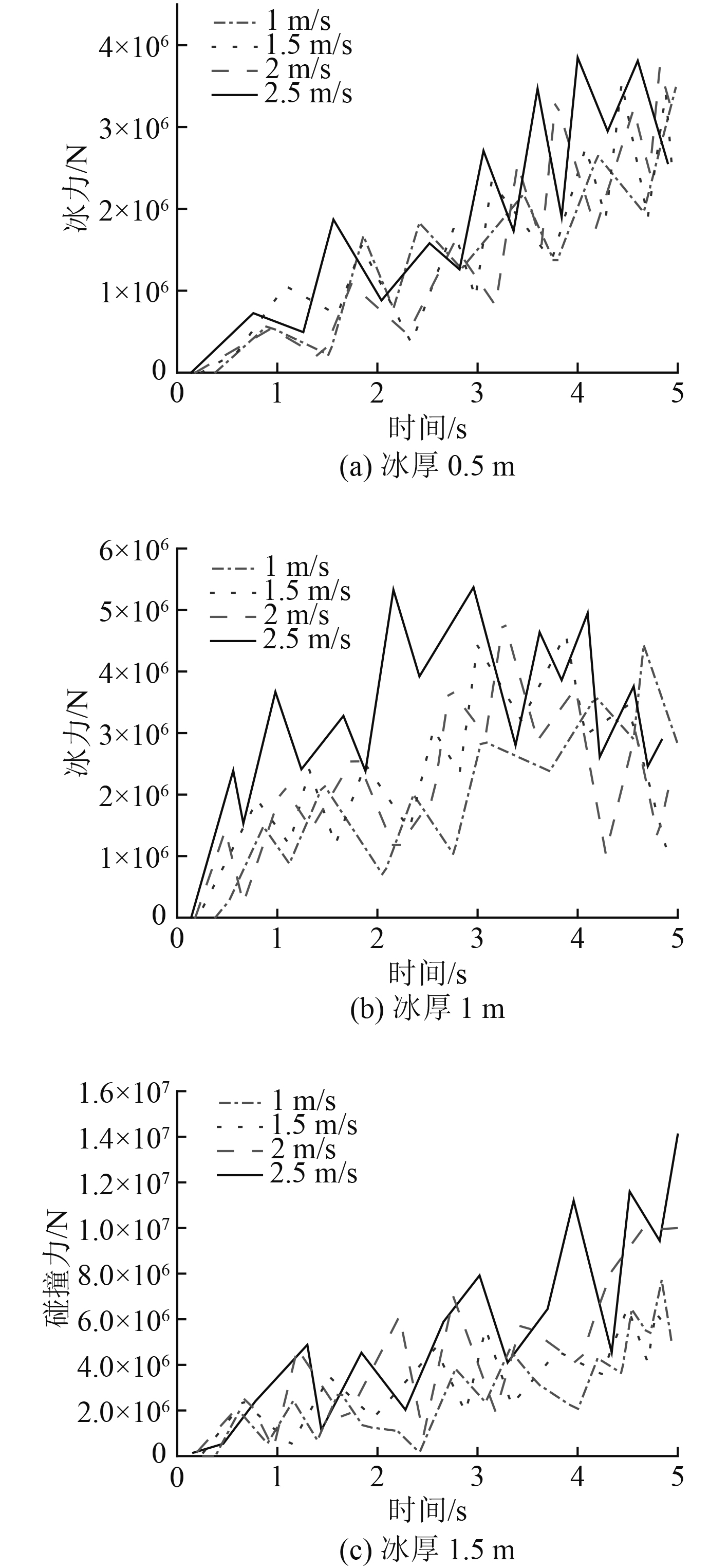图 4 不同船速冰厚下的冰力曲线 Fig. 4 Ice force curve under different ship speeds and ice thicknesses表 3 不同工况下冰力值 Tab.3 Ice force values under different working conditions

3 冰阻力经验模型 3.1 Lindqvist公式

 ${R_{ice}} = \left( {{R_c} + {R_b}} \right)\left( {1 + 1.4\frac{V}{{\sqrt {g{h_i}} }}} \right) + {R_s}\left( {1 + 9.4\frac{V}{{\sqrt {gL} }}} \right){\text{，}}$ (1)
 ${R_c} = 0.5{\sigma _f}h_i^2\frac{{\tan \phi + \mu \cos \phi /\cos \psi }}{{1 - \mu \sin \phi /\cos \psi }}{\text{，}}$ (2)
 $\begin{split} {R_b} =& \frac{{27}}{{64}}{\sigma _f}B\frac{{{h_i}^{1.5}}}{{\sqrt {\dfrac{E}{{12(1 - {\nu ^2})g{\rho _w}}}} }}\frac{{\tan \psi + \mu \cos \phi }}{{\cos \psi \sin \alpha }}\times\\ & \left(1 + \frac{1}{{\cos \psi }}\right){\text{，}} \end{split}$ (3)
 ${R_s} = ({\rho _w} - {\rho _i})g{h_i}B\left(T\frac{{B + T}}{{B + 2T}} + k\right){\text{，}}$ (4)
 $\begin{split} & k = \mu \biggr(0.7L - \frac{T}{{\tan \phi }} - \frac{B}{{4\tan \alpha }}+ \\ & T\cos \phi \cos \psi \sqrt {\frac{1}{{\sin {\phi ^2}}} + \frac{1}{{\tan {\alpha ^2}}}} \biggr){\text{，}} \end{split}$ (5)
 $\psi = \arctan \left( {\frac{{\tan \phi }}{{\sin \alpha }}} \right){\text{。}}$ (6)

3.2 Riska公式

 ${R_{ice}} = {C_1} + {C_2}V{\text{，}}$ (7)
 $\begin{split} {C_1} =& {f_1}\frac{1}{{\dfrac{{2T}}{B} + 1}}B{L_{par}}{h_i} + (1 + 0.021\phi ) \times\\ & ({f_2}Bh_i^2 + {f_3}{L_{bow}}h_i^2 + {f_4}B{L_{bow}}{h_i}){\text{，}} \end{split}$ (8)
 $\begin{split} {C_2} =& (1 + 0.063\phi )({g_i}h_i^{1.5} + {g_2}b{h_i}) +\\ & {g_3}{h_i}(1 + 1.2T/B)\frac{{{B^2}}}{{\sqrt L }}{\text{。}} \end{split}$ (9)表 4 Riska模型经验系数 Tab.4 Empirical coefficient of Riska model
3.3 Jeong公式

 $\begin{split} {R_i} =& 13.14{V^2} + {C_B}\Delta \rho g{h_i}BT + {C_C}F_h^{ - \alpha }{\rho _i}B{h_i}{V^2}+ \\ & {C_{BR}}S_N^{ - \beta }{\rho _i}B{h_i}{V^2}{\text{，}} \end{split}$ (10)
 ${F_h} = \frac{V}{{\sqrt {g{h_i}} }}{\text{，}}\;\;\;\;{S_N} = \frac{V}{{\sqrt {\dfrac{{{\sigma _f}{h_i}}}{{{\rho _i}B}}} }}{\text{。}}$ (11)表 5 各经验系数的取值 Tab.5 values of each empirical coefficient
3.4 Edwards公式

Edwards等通过模型和实船试验结果推导，并对之前的经验公式重新进行分析和改进，得到下式：

 $R = {\rho _w} \cdot B \cdot g \cdot {h_i}^2 \cdot \left(4.24 + 0.05 \cdot \frac{{{\sigma _f}}}{{{\rho _w} \cdot g \cdot {h_i}}} + 8.9{F_n}\right){\text{。}}$ (12)

4 计算结果分析比较 4.1 数值仿真与经验公式对比分析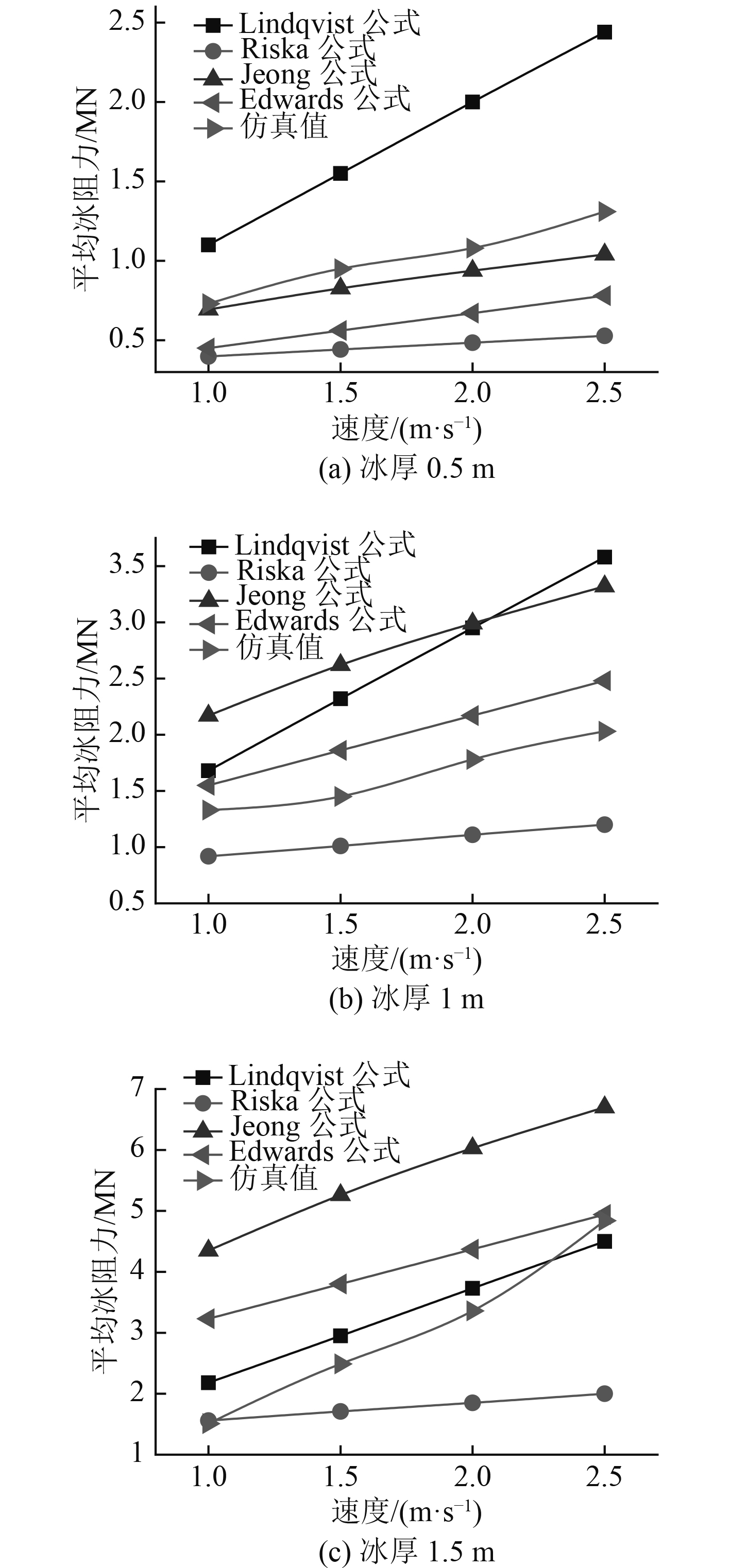图 5 不同工况下经验公式与数值模拟的冰力比较 Fig. 5 Comparison of empirical formula and numerical simulation results under different conditions

4.2 参数敏感性分析表 6 相关主要参数 Tab.6 Related main parameters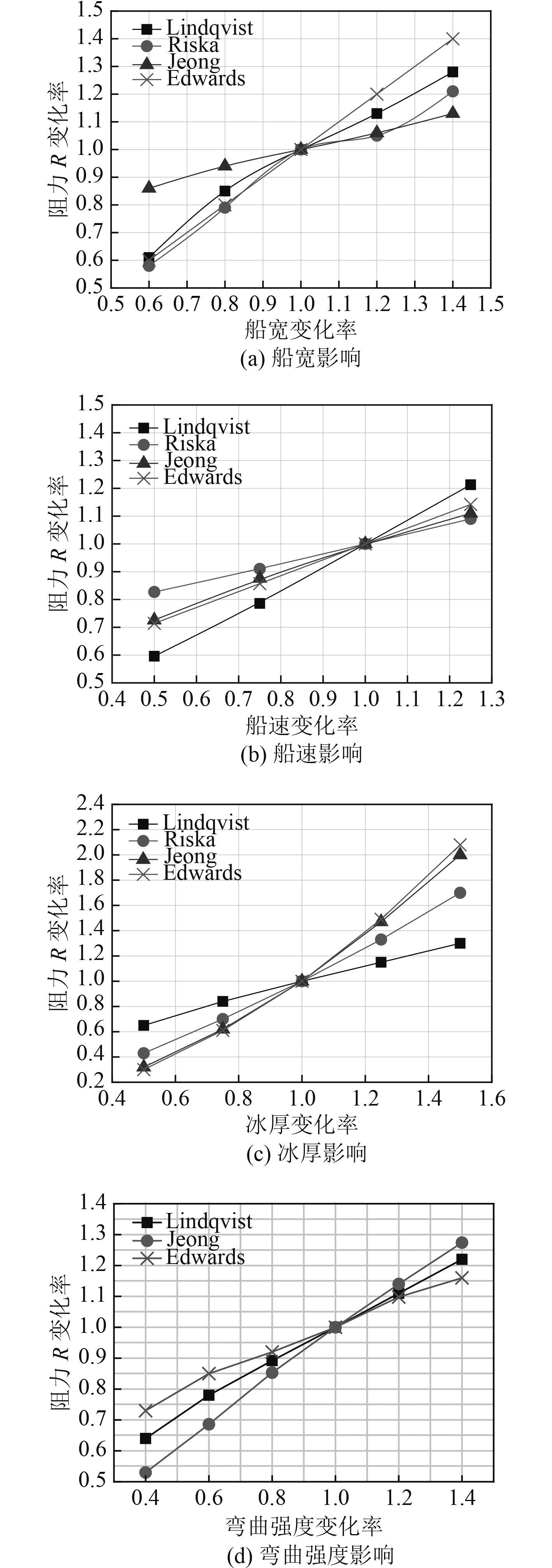图 6 参数敏感性分析 Fig. 6 Parameter sensitivity analysis

5 结　语

1）在不同应变率下，由钢板与冰锥碰撞仿真计算所得的冰力-位移曲线与文献中的实验数据结果基本吻合，说明该海冰材料模型适用于破冰过程的数值模拟。

2）由数值仿真和各种经验公式计算结果，可以发现，在不同船速和冰厚条件下，冰阻力均随船速和冰厚的增大而增大，而在船速和冰厚2个参数中，冰厚对冰阻力影响更大。

3）经与数值仿真结果对比分析，不同的经验公式适用于不同冰厚条件下的冰阻力预报：冰厚为0.5 m时，数值仿真结果与Jeong公式计算值最为接近；冰厚为1 m时，数值仿真结果与Edwards公式计算值最为接近；冰厚为1.5 m时，数值仿真结果与Lindqvist公式的计算值最为接近，可以为进行快速冰阻力预报时选取适合的经验公式提供依据。

4）对不同经验公式中的不同参数进行敏感性分析，反映出了冰阻力随各参数变化率增大而增大的变化趋势，但船宽、船速、冰厚以及冰弯曲强度等参数对各经验公式冰阻力计算结果的敏感性也各有不同。船宽和冰厚的变化对Edwards公式影响最大，船速对Lindqvist公式影响最大，可以为船体结构设计提供参考。

  韩端锋, 乔岳, 等. 冰区航行船舶冰阻力研究方法综述[J]. 船舶力学, 2017, 21(8): 41-54. HAN Duan-feng, QIAO Yue, et al. A review of ice resistance research methods for ice-going ships[J]. Journal of Ship Mechanics, 2017, 21(8): 41-54.  LINDQVIST G. A straightforward method for calculation of ice resistance of ships[C]// Proceedings of the TenthInternational Conference on Port and Ocean Engineering under Arctic Conditions. Lulea, Sweden, 1989: 722-735.  RISKA K, WILHELMSON M, ENGLUND K, LEIVISKA T. Performance of merchant vessels in the Baltic[R]. Winter Navigation Research Board. Helsinki, Finland, 1997.  JEONG S Y, LEE C J, CHO S R. Ice resistance prediction for standard icebreaker model ship[C]// Proceedings of the Twentieth (2010) International Offshore and Polar Engineering Conference. Beijing, China, 2010: 1300-1304.  EDWARDS R Y, MAJOR R A. Influence of major characteristics of icebreaker hulls on their powering requirements and maneuverability in ice[R], 1976.  任奕舟, 邹早建. 破冰船在冰层中连续破冰过程的数值模拟[J]. 振动与冲击, 2016, 35(18): 210-223. REN Yi-zhou, ZOU Zao-jian. Numerical simulation of the continuous icebreaking by an icebreaker in level ice[J]. Jouranal of Vibration and Shock, 2016, 35(18): 210-223.  刁峰, 陈京普, 等. 极地船舶冰阻力经验模型研究[J]. 中国造船, 2016, 57(2): 38-44. DIAO Feng, CHEN Jing-pu, et al. Study on ice resistance estimation using empirical models for polar ships[J]. Shipbuilding of China, 2016, 57(2): 38-44. DOI:10.3969/j.issn.1000-4882.2016.02.005  JIAN Hu, LI Zhou. Further study on level ice resistance and channel resistance for an icebreaking vessel[J]. International Journal of Naval Architecture and Ocean Engineering, 2016, 8: 169-176. DOI:10.1016/j.ijnaoe.2016.01.004  DALEY C, KENDRICK A. Safe speed in ice [R]. Final report, prepared for BMT fleet technology, 2011 Reference: 6931DFR.Rev00, Ottawa, ON, Canada.  KIM M C. Study on icebreaking performance of the Korea icebreaker ARAON in the Arctic sea[J]. International Journal of Naval Architecture and Ocean Engineering, 2011, 3(3): 208-215. DOI:10.2478/IJNAOE-2013-0064  蔡毅, 邢岩, 胡丹. 敏感性分析综述[J]. 北京师范大学学报(自然科学版), 2008(1): 9-16. CAI Yi, XING Yan, HU Dan. On sensitivity anaysis[J]. Journal of Beijing Normal University (Natural Science), 2008(1): 9-16. DOI:10.3321/j.issn:0476-0301.2008.01.003